Create a new printable
Answer key also includes questions
Answer key only gives the answers
No answer keyMath Worksheets
Time

Sample - Click above to make a new math worksheet (PDF).
 Name _____________________________Date ___________________
Half-Hour
Write the time two ways.

1.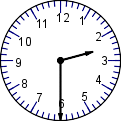half past ______ : ___
2.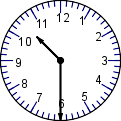half past ______ : ___
3.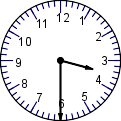half past ______ : ___
4.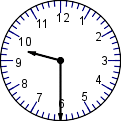half past ______ : ___
5.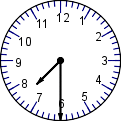half past ______ : ___
6.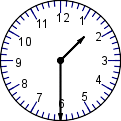half past ______ : ___
 7 * This is a pre-made sheet.Use the link at the top of the page for a printable page.
8.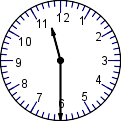half past ______ : ___
9.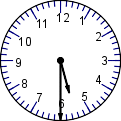half past ______ : ___

 Answer Key

Sample
This is only a sample worksheet.

Create a new printable
Answer key also includes questions
Answer key only gives the answers
No answer keyMath Worksheets
Time